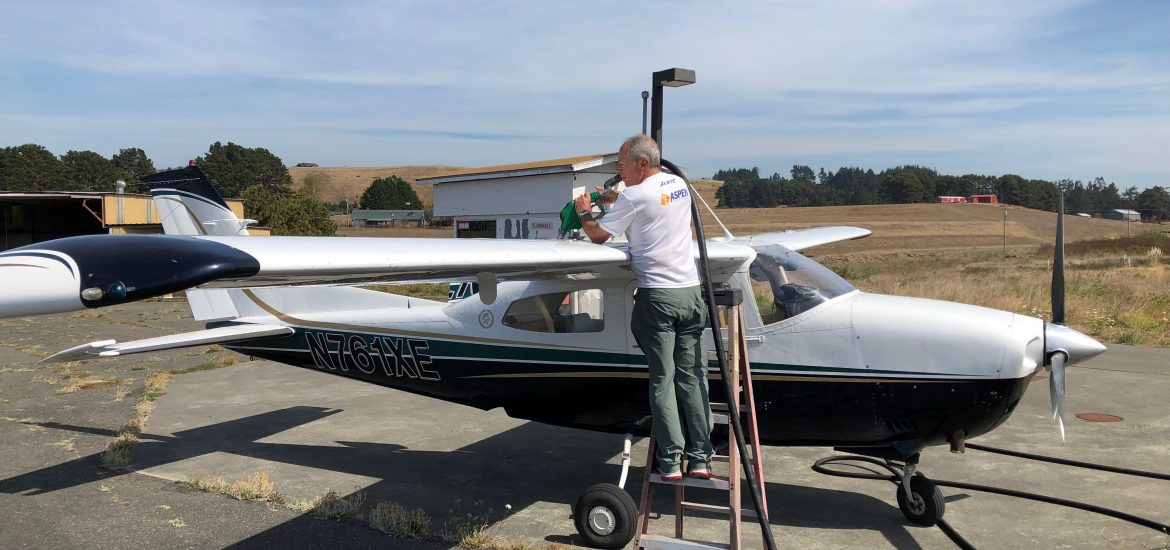# Plane Flight Mathematics### How far can a Cessna fly?

Use these assumptions:

• Cessna plane carries 100 gallons of fuel
• Cessna plane gets 5 miles/gallon
• Fuel costs \$6/gallonOn a Cessna flight from an airport in Humboldt County I was inspired to develop a plane flight mathematics lesson — how far can a Cessna fly?

## Common Core Math Standards – 4th

#### Operations and Algebraic Thinking

Use the four operations with whole numbers to solve problems

3. Solve multistep word problems posed with whole numbers and having whole-number answers using the four operations, including problems in which remainders must be interpreted. Represent these problems using equations with a letter standing for the unknown quantity. Assess the reasonableness of answers using mental computation and estimation strategies including rounding.

Generate and analyze patterns.

5. Generate a number or shape pattern that follows a given rule. Identify apparent features of the pattern that were not explicit in the rule itself. For example, given the rule “Add 3” and the starting number 1, generate terms in the resulting sequence and observe that the terms appear to alternate between odd and even numbers. Explain informally why the numbers will continue to alternate in this way.

#### Number and Operations in Base Ten

Generalize place value understanding for multi-digit whole numbers.

1. Recognize that in a multi-digit whole number, a digit in one place represents ten times what it represents in the place to its right. For example, recognize that 700 ÷ 70 = 10 by applying concepts of place value and division.

2. Read and write multi-digit whole numbers using base-ten numerals, number names, and expanded form. Compare two multidigit numbers based on meanings of the digits in each place, using >, =, and < symbols to record the results of comparisons.

3. Use place value understanding to round multi-digit whole numbers to any place. Use place value understanding and properties of operations to perform multi-digit arithmetic. 4. Fluently add and subtract multi-digit whole numbers using the standard algorithm.

#### Measurement and Data

2. Use the four operations to solve word problems involving distances, intervals of time, liquid volumes, masses of objects, and money, including problems involving simple fractions or decimals, and problems that require expressing measurements given in a larger unit in terms of a smaller unit. Represent measurement quantities using diagrams such as number line diagrams that feature a measurement scale